# CBSE Class 12 Physics Notes Chapter 1 Electric Charges and Fields

Have you ever heard a crackle or seen a spark while taking off your synthetic clothes or sweater, especially in dry weather? How about some explanation about this phenomenon? Another example of electric discharge is the lightning that we see in the sky during thunderstorms. All these experiences are the result of the discharge of electric charges through your body that accumulated due to the rubbing of insulating surfaces. It is also caused due to the generation of static electricity. CBSE Class 12 Physics Notes Chapter 1 Electric Charges and Fields discusses these facts in detail. Electrostatics deals with the study of forces, fields and potentials arising from static charges.

#### For more information on Charging by Induction, watch the below video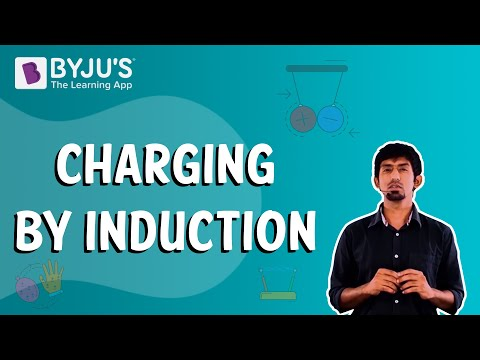### Electric Charge

The term ‘electricity’ is derived from Elektron, a Greek word meaning amber. The properties of matter, atoms and molecules are determined by the magnetic and electric forces present in them. There are also only 2 kinds of an entity called the electric charge.

An experiment conducted also suggested that there are two kinds of electrification wherein (i) like charges repel and (ii) unlike charges attract each other. The property that differentiates these 2 kinds of charges is called the polarity of charge.

### Conductors and Insulators

When an experiment was conducted on electric charges due to frictional electricity, it was found that conductors assist in the movement of electric charge, but insulators do not behave in the same manner. Metal, Earth, and human bodies are all examples of conductors, while porcelain, nylon, and wood all offer high resistance to the passage of electricity through them as they are insulators.

#### What Are the Properties of Electric Charge?

An electric charge has three fundamental properties:

• Quantization- This property states that the total charge of a body represents the integral multiple of a basic quantum of charge.
• Additive- This property of electric charges represents the total charge of a body as the algebraic sum of all the singular charges acting on the system.
• Conservation- This property states that the total charge of a system remains unaffected by time. In other words, when objects get charged due to friction, a transfer of charge from one object to another occurs. Charges can neither be created nor destroyed.

### Coulomb’s Law

Coulomb’s law states that the mutual electrostatic force existing between two point charges, A and B, is proportional to their product which is AB and inversely proportional to the square of the distance between them (

$$\begin{array}{l}r_{AB}\end{array}$$
). The equation is
$$\begin{array}{l}F_{BA}= force\, on\, B\, due \, to \, A = k(AB)\div r_{AB}^{2}\end{array}$$

Mathematically,

This law consists of constant terms, which are also called a constant of proportionality and are represented by ‘k’, and its values are

$$\begin{array}{l}k=9 * 10^{9} Nm^{2}C^{-2}\end{array}$$
$$\begin{array}{l}Nm^{2}C^{-2}\end{array}$$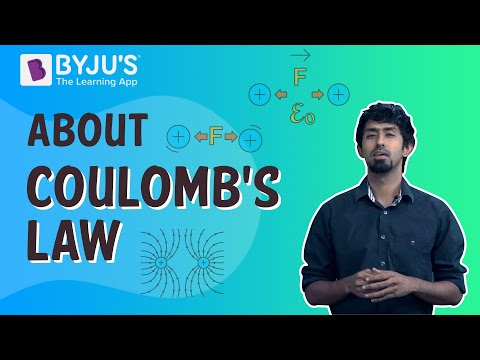### Forces between Multiple Charges

Even if the mutual electric force between two charges is given by Columb’s law, it does not help to calculate the force on a charge where there is not one but several charges around.  It has been proved via an experiment that force on any charge due to a number of other charges is the vector sum of all the forces on that charge due to the other charges, taken one at a time.

#### Superposition Principles

According to the superposition principle, the property of two charges to repel and attract each other remains unaffected even though there is the presence of a third additional charge.

Consider q1, q2 and q3 as three charges of a system. Here, if the force on q1 due to qis denoted by F12.

Then, $F_{12}=\frac{1}{4\pi \varepsilon}\frac{q_{1}q_{2}}{r^{2}_{12}}r_{12}$

Likewise, the force on qdue to q3,  denoted by F13 is $F_{13}=\frac{1}{4\pi \varepsilon}\frac{q_{1}q_{3}}{r^{2}_{13}}r_{13}$

Thus, the total force Fon qdue to 2 charges q2 and qis  $F_{1}=F_{12}+F_{13}$

$F_{1}=\frac{1}{4\pi \varepsilon}\frac{q_{1}q_{2}}{r^{2}_{12}}r_{12}+\frac{1}{4\pi \varepsilon}\frac{q_{1}q_{3}}{r^{2}_{13}}r_{13}$

### Properties of Electric Field Lines

Some of the general properties of field lines are:

• Field lines show a continuous curve without having any breakage in a charge-free region.
• Two-line never cross each other.
• These electric field lines start on the positive charge and end on the negative charge.
• Electrostatic field lines do not form any closed loops.

### Electric Flux

The total number of electric field lines passing a given area in a unit of time is defined as the electric flux. However, we note that there is no flow of a physically observable quantity like in the case of liquid flow. Coming to the definition,  Electric flux  Δθ through an area element ΔS is defined by

Δθ= E.ΔS= E ΔS  cosθ

This is proportional to the number of field lines cutting the area element. The angle θ here is the angle between E and ∆S. In a closed surface, where the convention is already stated, θ is the angle between E and the outward normal to the area element. To calculate the total flux through any given surface, divide the surface into small area elements, calculate the flux at each element and add them up. Thus, the total flux θ through a surface S is θ ~ Σ E. ∆S. The approximation symbol is used because the electric field E is taken to be constant over the small area element.

#### For more information on Electric Flux and Electric Force, watch the below videos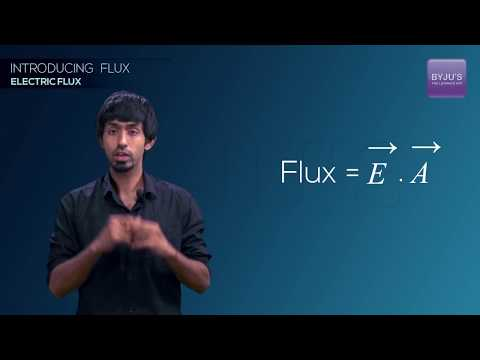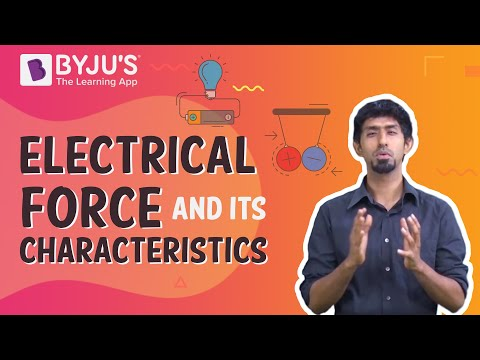### Electric Dipole

It is a pair of equal or opposite charges, A and -B, which are separated by distance 2x. The dipole moment vector (let’s assume it is p) has a magnitude 2Ax and is in the direction of the dipole axis from -B to A

#### For more information on Flux through a Sphere, watch the below video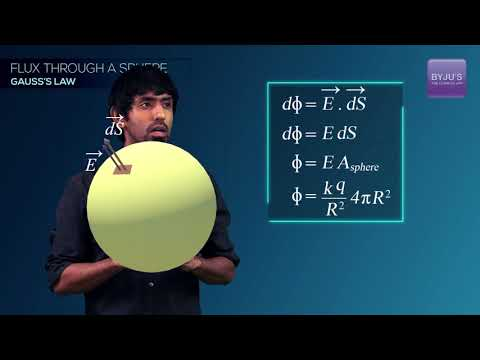To know more about Electric Charge and Electric Field, keep visiting BYJU’S website.

### Important Question

1. (a) The Statement needs an explanation ‘electric charge of a body is quantized’.

(b) Why can one ignore the quantization of electric charge when dealing with macroscopic, i.e., large-scale charges?

2. Explain how the law of conservation of charge works on this phenomenon when charges appear on both the silk cloth and the glass rod when they are rubbed together.?

(a)Why electrostatic field line is not a continuous curve and cannot have sudden breaks?
(b) Why do two field lines never cross each other at any point?

Related Topics:

NCERT Solutions for Electric Charges and Fields

NCERT Exemplars for Electric Charges and Fields

Electric Charge and Field Important Questions

## Frequently Asked Questions on CBSE Class 12 Physics Notes Chapter 1 Electric Charges and Fields

Q1

### What is meant by induction?

Induction is the property of a conductor to become electrified when there is the presence of a charged body present nearby.

Q2

### What does Coulomb’s Law state?

Coulomb’s Law: The force of attraction or repulsion acting along a straight line between two electric charges is directly proportional to the product of the charges and inversely to the square of the distance between them.

Q3

### What is electric flux?

The measure of a count of the number of electric field lines crossing an area is known as electric flux.